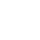CurrentHome>Resources
Third-order IM Distortion Measurement of S3302 Handheld Spectrum Analyzer
Release time :2021.03.14 Reading quantity:46 source:Salukirf, original

Saluki S3302 series handheld spectrum analyzer has a variety of measurement functions. One of the measurement methods is the third-order IM distortion measurement. This section we will introduce this measurement.

1. Definition of Third-order IM Distortion

Mutual interference between equipment in common in crowd operating environment of the communications system. For example, second-order and third-order IM distortion is common in narrow-band systems. When there are two signals (F1 and F2) in one system, they and second harmonic distortion signals generated by them (2F1 and 2F2) mix and become third-order IM products 2F2-F1 and 2F1-F2 very close to original signals, thus resulting in high-order IM distortion. Such distortion products are mostly generated by devices such as amplifiers and mixers in the system.

The measurement of third-order IM distortion is described below. This section provides an example of how to display two signals at the same time on the screen of the spectrum analyzer, and introduces how to set the resolution bandwidth, mixer level and reference level, as well as some marker functions.

2. Measurement Procedures

a) Connect the tested instrument with spectrum analyzer as shown in Fig. 2.

This example involves one 6dB directional coupler, one 1GHz signal generator and one 1.001GHz signal generator. Of course, the signal generator of other frequencies are allowed. However, the frequency interval must be approximately 1MHz in this example.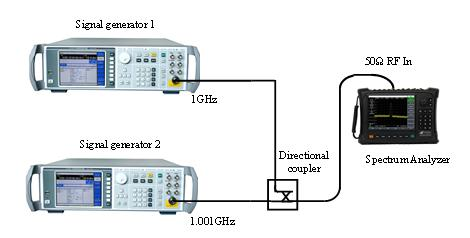Fig. 2 Connection of Third-order IM Distortion Measurement System

Set the output frequency of one signal generator as 1GHz and the output frequency of the other signal generator as 1.001GHz, so that the frequency interval of two signals transmitted into the spectrum analyzer is 1MHz.

Set the same output amplitude for both signal generators (-20dBm in this example).

b) Set the spectrum analyzer until both signals are displayed on its screen at the same time.

Press [Reset].

Press [Frequency], [Center Frequency], 1.0005[GHz].

Press [frequency], [Span] and 5[MHz].

You can see that both signals are at the center of the screen, as shown in Fig.3. If the applied frequency interval is different from that in this example, select the span more than three times of the frequency interval of the signal generators.

c) Reduce the resolution bandwidth until you can see the distortion product.

Press [BW] and reduce the resolution bandwidth with the step key [↓].

d) Adjust both signal generators until the amplitudes of input signals are the same.

Press [Maker], [Delta], [Peak] and [Next Peak]. Adjust the signal generator corresponding to the marker until the amplitude difference is zero. If required, reduce the video bandwidth.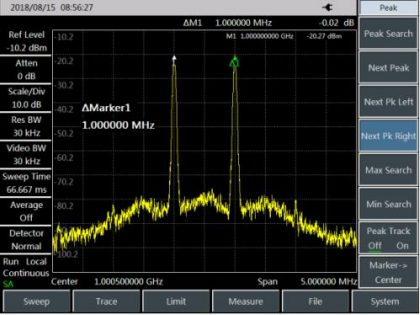Fig. 3 Signals at Center of Display of Spectrum Analyzer

e) Set the reference level and make the signal peak at the reference level:

Press [Peak] and [Peak Search], and read the peak power.

Press [Amplitude], [Reference Level].

In order to achieve the best measurement accuracy, the signal peak of the signal generators should be set at the reference level, as shown in Fig. 4.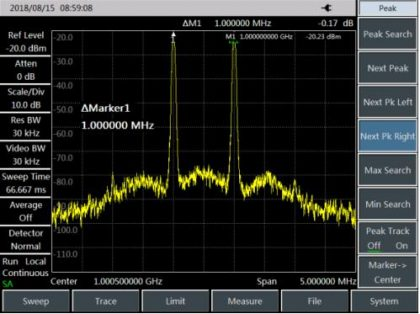Fig. 4 Signal Peak at Reference Level

f) Set the second marker and measure the distortion product:

Once the marker is activated, the second marker will be generated by the differential marker function, and the difference between both markers will be displayed. In this case, relative measurement can be performed easily.

Press [Peak] to activate one marker.

Press [Maker] and [Delta] to activate the second marker.

Press [Peak] and [Next Pk Left] or [Next Pk Right] to set the second marker at the peak point of the distortion product beside the signal generated by the signal generator. As shown in Fig.5, the frequency and amplitude difference of both markers will be shown in the marker display zone, and the marker amplitude difference will be the measured value of third-order IM distortion.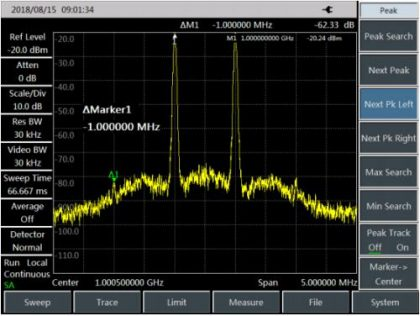Fig. 5 Relative Measurement of Internal Modulation Distortion

g) Calculation of third-order interception (TOI) point:

The ratio (in dB) of the distortion component level to signal level has no great significance for system distortion, unless the signal level is specified. The interception point can be applied to specify and evaluate the system distortion level. The difference between the third-order distortion component level and fundamental signal level is twice of that between the fundamental signal level and third-order interception point. The third-order interception (TOI) point can be calculated by the following formula: